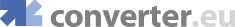online velocity conversions, online velocity angular conversions, degree/second, radian/second
Engineering converters
 Acceleration - Angular Acceleration - Linear Angle Area Data Storage Data Transfer Density Energy Force Moment of Inertia Numbers Power Pressure Specific Volume Time Torque Velocity - Angular
Heat converters
Fluids converters
Light converters
Electricity converters
Magnetism converters
Other converters

 Show currency exchange rate: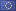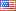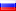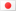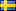… Get a Converter API tool for your website for free!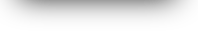All Velocity - Angular Conversion
Convert fromConvert to
°/s =  °/s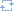Change direction